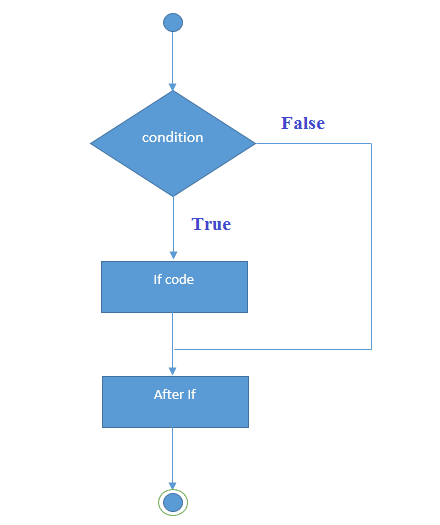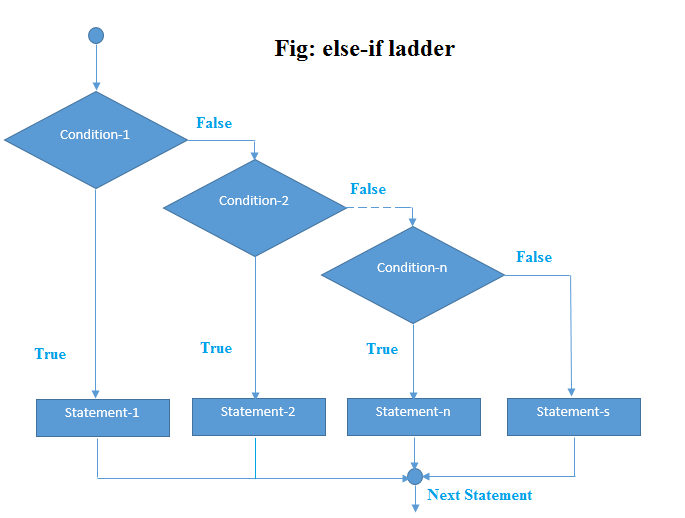# Java Tutorial

## Java If-else Statement

##### Decision Making in Java (if, if-else, switch, break, continue, jump)

Decision Making in programming is similar to decision making in real life. In programming also we face some situations where we want a certain block of code to be executed when some condition is fulfilled.

A programming language uses control statements to control the flow of execution of program based on certain conditions. These are used to cause the flow of execution to advance and branch based on changes to the state of a program.

The Java if statement is used to test the condition.There are various types of if statement in java.

• if statement
• if-else statement
• nested if statement
##### if statement

if statement is the most simple decision making statement. It is used to decide whether a certain statement or block of statements will be executed or not i.e if a certain condition is true then a block of statement is executed otherwise not.

##### Syntax:
```
if(condition)
{
// Statements to execute if
// condition is true
}
```##### Example:
```
public class IfExample
{
public static void main(String[] args)
{
int age=31;
if(age>18){
System.out.print("Age is greater than 18");
}
}
}

```

##### Output:
```

Age is greater than 18

```
```
import java.util.Scanner;
public class IfExample
{
public static void main(String[] args)
{
int age;
Scanner p=new Scanner(System.in);
age=p.nextInt();
if(age>18){
System.out.print("Age is greater than 18");

}
}
}
```

##### Output:
```

Age is greater than 18

```
##### Java if-else Statement

The Java if-else statement also tests the condition. It executes the if block if condition is true otherwise else block is executed.

##### Syntax:
```
if(condition)
{
//code if condition is true
}else
{
//code if condition is false
}
```##### Java Program to Find the Largest Two Numbers
```
public class IfExample
{
public static void main(String[] args)
{
int num1=31,num2=36;
if(num1>num2)
{
System.out.println(+num1+" is large");
}
else
{
System.out.println(+num2+" is large");
}
}
}

```

```
36 is large

```
##### Java Program to Find the Largest Two Numbers
```
import java.util.Scanner;
public class IfExample
{
public static void main(String[] args)
{
int num1,num2;
Scanner p=new Scanner(System.in);
System.out.print("Enter first value:");
num1=p.nextInt();
System.out.print("Enter second value:");
num2=p.nextInt();
if(num1>num2)
{
System.out.println(+num1+" is large");
}
else
{
System.out.println(+num2+" is large");
}
}
}

```

##### Output:
```
Enter first value:31
Enter second value:36
36 is large

```

Here, a user can decide among multiple options.The if statements are executed from the top down. As soon as one of the conditions controlling the if is true, the statement associated with that if is executed, and the rest of the ladder is bypassed. If none of the conditions is true, then the final else statement will be executed.

##### Syntax:
```
if (condition1)
statement1;
else if (condition2)
statement2;
else if (condition3)
statement3;
.
.
else
statementn;
```##### Example:
```
// Java program to illustrate if-else-if ladder
class ifelseifDemo
{
public static void main(String args[])
{
int i = 50;

if (i == 10)
System.out.println("i is 10");
else if (i == 20)
System.out.println("i is 20");
else if (i == 30)
System.out.println("i is 30");
else if (i == 40)
System.out.println("i is 40");
else if (i == 50)
System.out.println("i is 50");
else if (i == 70)
System.out.println("i is 70");
else if (i == 80)
System.out.println("i is 80");
else
System.out.println("i is not present");
}
}

```

##### Output:
```

i is 50

```
```
import java.util.Scanner;
class ifelseifDemo
{
public static void main(String args[])
{
int i;
Scanner p=new Scanner(System.in);
System.out.print("Enter i value:");
i=p.nextInt();
if (i == 10)
System.out.println("i is 10");
else if (i == 20)
System.out.println("i is 20");
else if (i == 30)
System.out.println("i is 30");

else if (i == 40)
System.out.println("i is 40");

else if (i == 50)
System.out.println("i is 50");

else if (i == 70)
System.out.println("i is 70");
else if (i == 80)
System.out.println("i is 80");
else
System.out.println("i is not present");
}
}

```

```

Enter i value:30
i is 30

```
##### Java nested if statement

A nested if is an if statement that is the target of another if or else. Nested if statements means an if statement inside an if statement. Yes, java allows us to nest if statements within if statements. i.e, we can place an if statement inside another if statement.

##### Syntax:
```
if (condition1)
{
// Executes when condition1 is true
if (condition2)
{
// Executes when condition2 is true
}
}
```##### Example:
```
// Java program to illustrate nested-if statement
class NestedIfDemo
{
public static void main(String args[])
{
int i = 10;

if (i == 10)
{
// First if statement
if (i < 15)
System.out.println("i is smaller than 15");

// Nested - if statement
// Will only be executed if statement above
// it is true
if (i < 12)
System.out.println("i is smaller than 12 too");
else
System.out.println("i is greater than 15");
}
}
}

```

##### Output:
```

i is smaller than 15
i is smaller than 12 too

```
```
import java.util.Scanner;
// Java program to illustrate nested-if statement
class NestedIfDemo
{
public static void main(String args[])
{
int i;
Scanner p=new Scanner(System.in);
System.out.print("Enter i value:");
i=p.nextInt();
if (i == 10)
{
// First if statement
if (i < 15)
System.out.println("i is smaller than 15");

// Nested - if statement
// Will only be executed if statement above
// it is true
if (i < 12)
System.out.println("i is smaller than 12 too");
else
System.out.println("i is greater than 15");
}
}
}

```

##### Output:
```

Enter i value:10
i is smaller than 15
i is smaller than 12 too

```
##### Example for Nested If in java Programming
```
import java.util.Scanner;
class NestedifExample
{
public static void main(String[] args)
{
/* Example for Nested If in java Programming */
int age;
Scanner p=new Scanner(System.in);
age=p.nextInt();

if ( age < 18 )
{
System.out.println("You are Minor.");
System.out.println("Not Eligible to Work");
}
else
{

if (age >= 18 && age <= 60 )
{
System.out.println("You are Eligible to Work ");
}
else
{
System.out.println("You are too old to work as per the Government rules");
}
}
}
}
```

##### Output:
```
15
You are Minor.
Not Eligible to Work